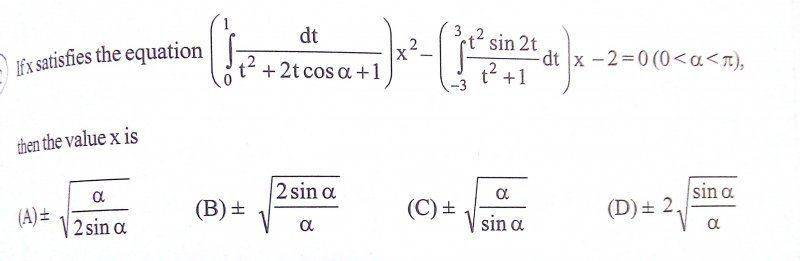# Definite integration problem

## Homework Statement

∫dt/(t^2 +2tcos a + 1)
(Limits of the integral are from 0 to 1)
(0<a<π)

## The Attempt at a Solution

Put t=sin a
dt=cosa da
∫dt/(t^2 +2tcos a + 1) = ∫cos a da/(sin^2 a + sin 2a + 1) [ limits of integration changed to 0 to π/2]
= ((cosec a)/2) ∫sin 2a da/(sin^2 a + sin 2a + 1)

Last edited by a moderator:

Mark44
Mentor

## Homework Statement

∫dt/(t^2 +2tcos a + 1)
(Limits of the integral are from 0 to 1)
(0<a<π)

## The Attempt at a Solution

Put t=sin a
dt=cosa da
∫dt/(t^2 +2tcos a + 1) = ∫cos a da/(sin^2 a + sin 2a + 1) [ limits of integration changed to 0 to π/2]
= ((cosec a)/2) ∫sin 2a da/(sin^2 a + sin 2a + 1)
I don't know what you did in this last step, but you can't bring csc(a) out as if it were a constant.
utkarsh009 said: